# Using quantile regression to evaluate police response times

Jeff Asher recently had a post on analysis of response times across many agencies. One nitpick though (and ditto for prior analyses I have seen, such as Scott Mourtgos and company), is that you should not use linear models (or means in general) to describe response time distributions. They are very heavily right skewed, and so the mean tends to be not representative of the bulk of the data.

When evaluating changes in response time, imagine two simplistic scenarios. One, every single call increases by 5 minutes, so what used to be 5 is now 10, 20 is now 25, 60 is now 65, etc. That is probably not realistic for response times, it is probably calls in the tail (ones that take a very long time to wait for an opening in the queue) are what changes. E.g. 5 is still 5, 20 is still 20, but 60 is now 120. In the latter scenario, the left tail of the distribution does not change, only the right tail. In both scenarios the mean shifts.

I think a natural way to model the problem is instead of focusing on means, is to use quantile regression. It allows you to generalize the entire distribution (look at the left and right tails) and still control for individual level circumstances. Additionally, often emergency agencies set goals along the lines of “I want to respond to 90% of emergency events with X minutes”. Quantile regression is a great tool to describe that 90% make. Here I am going to show an example using the New Orleans calls for service data and python.

First, we can download the data right inside of python without saving it directly to disk. I am going to be showing off estimating quantile regression with the `statsmodel` library. I do the analysis for 19 through 22, but NOLA has calls for service going back to the early 2010s if folks are interested.

``````import pandas as pd
import statsmodels.formula.api as smf

yr_url = [y19,y20,y21,y22]
res_pd = [pd.read_csv(url) for url in yr_url]
data = pd.concat(res_pd,axis=0) # alittle over 1.7 million``````

Now we do some data munging. Here I eliminate self initiated events, as well as those with missing data. There then are just a handful of cases that have 0 minute arrivals, which to be consistent with Jeff’s post I also eliminate. I create a variable, `minutes`, that is the minutes between the time created and the time arrived on scene (not cleared).

``````# Prepping data
data = data[data['SelfInitiated'] == 'N'].copy() # no self init
data = data[~data['TimeArrive'].isna()].copy()   # some missing arrive
data['begin'] = pd.to_datetime(data['TimeCreate'])
data['end'] = pd.to_datetime(data['TimeArrive'])
dif = data['end'] - data['begin']
data['minutes'] = dif.dt.seconds/60
data = data[data['minutes'] > 0].copy() # just a few left over 0s

# Lets look at the distribution
data['minutes'].quantile([0.1,0.2,0.3,0.4,0.5,0.6,0.7,0.8,0.9])``````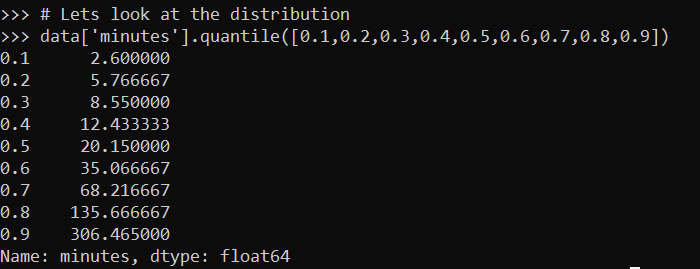For quantiles, for the entire sample the median time is around 20 minutes, the 10th percentile is under 3 minutes and the 90th percentile is around 5 hours. Using the mean here (which in Jeff’s post varies from 50 to 146 minutes over the same 4 year period), can be somewhat misleading.

An important component of response times is differentiating between different priority calls. NOLA in their data, higher numbers are higher priority. Zero priority are things NOLA says don’t necessarily need an officer at all. So it could be those “0 priority” calls are really just dragging the overall average down over time, although they may have little to do with clearance rates or public safety overall. The priority category fields also has sub-categories, e.g. 1A is higher priority than 1B. To keep the post simple I just breakdown by integer leading values, not the sub letter-categories.

``````# Priority just do 1/2/3
# 3 is highest priority
data['PriorCat'] = data['Priority'].str
# Only 5 cases of 3s, will eliminate these as well
data.groupby('PriorCat')['minutes'].describe()``````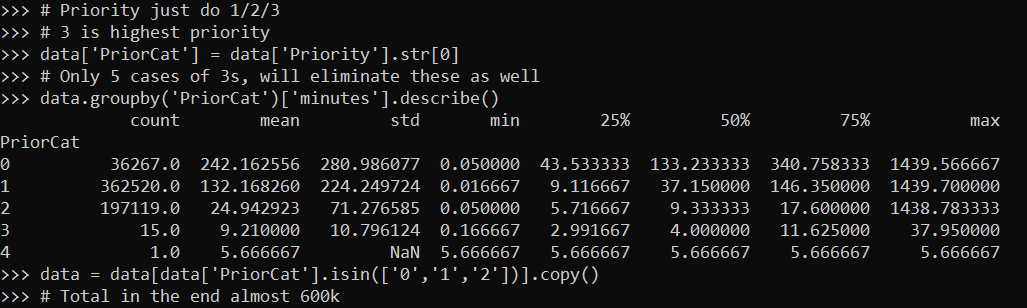Here you can really see the right skewness – priority 2 calls the mean is 25 minutes, but the median is under 10 minutes for the entire sample. A benefit of quantile regression I will use in a bit, the few outlying cases (beyond the quantiles of interest), really don’t impact the analysis. So those cases that take almost 24 hours (I imagine they are just auto-filled in like that in the data), really don’t impact estimates of smaller quantiles. But they can have noticeable influence on mean estimates.

Some final data munging, to further simplify I drop the 16 cases of priority 3s and 4s, and add in a few more categorical covariates for hour of the day, and look at months over time as categorical. (These decisions are more so to make the results easier to parse in a blog post in simpler tables, it would take more work to model a non-linear continuous over time variable, say via a spline, and make a reasonable ordinal encoding for the sub-priority categories.)

``````# only worry about 0/1/2s
data = data[data['PriorCat'].isin(['0','1','2'])].copy()
# Total in the end almost 600k cases

# Some factor date variables
def dummy_stats(vdate,begin_date):
bd = pd.to_datetime(begin_date)
year = vdate.dt.year
month = vdate.dt.month
week_day = vdate.dt.dayofweek
hour = vdate.dt.hour
diff_days = (vdate - bd).dt.days
# if binary, turn week/month into dummy variables
return diff_days, week_day, hour, month, year

dn, wd, hr, mo, yr = dummy_stats(data['begin'],'1/1/2022')
data['Hour'] = hr
data['Month'] = mo
data['Year'] = yr

# Lets just look at months over time
data['MoYr'] = data['Year'] + data['Month']/100``````

Now finally onto the modeling stuff. For those familiar with regression, quantile regression instead of predicting the mean predicts a quantile of the distribution. Here I show predicting the 50th quantile (the median). For those not familiar with regression, this is not all that different than doing a pivot table/group by, but aggregating by quantiles instead of means. Regression is somewhat different than the simpler pivot table, since you “condition on” other continuous factors (here I “control for” hour of day), but in broad strokes is similar.

Here I use a patsy “R style” formula, and fit a categorical covariate for the 0/1/2 categories, hour of day, and the time varying months over time (to see the general trends). The subsequent regression table is big, so will show in parts:

``````# Quantile regression for median
mod = smf.quantreg("minutes ~ C(PriorCat, Treatment(reference='2')) + C(Hour) + C(MoYr)", data)
res50 = mod.fit(q=0.5)
res50.summary()``````

First, I use 2 priority events as the referent category, so you can see (in predicting the median of the distribution), priority 1 events have a median 24 minutes longer than priority 2, and priority 0 have a median two hours later. You can see some interesting patterns in the hour of the day effects (which are for the overall effects, not broken down by priority). So there are likely shift changes at 06:00, 14:00, and 22:00 that result in longer wait times.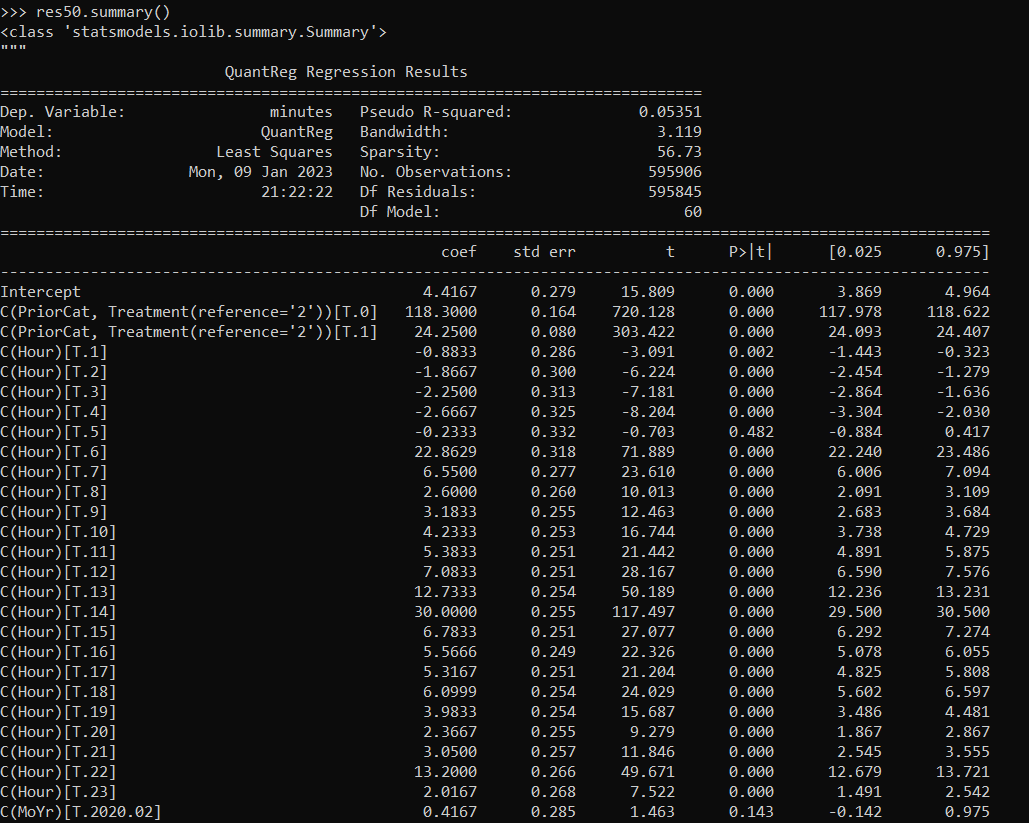But of most interest are patterns over time, here is the latter half of that table, showing median estimates over the months in this sample.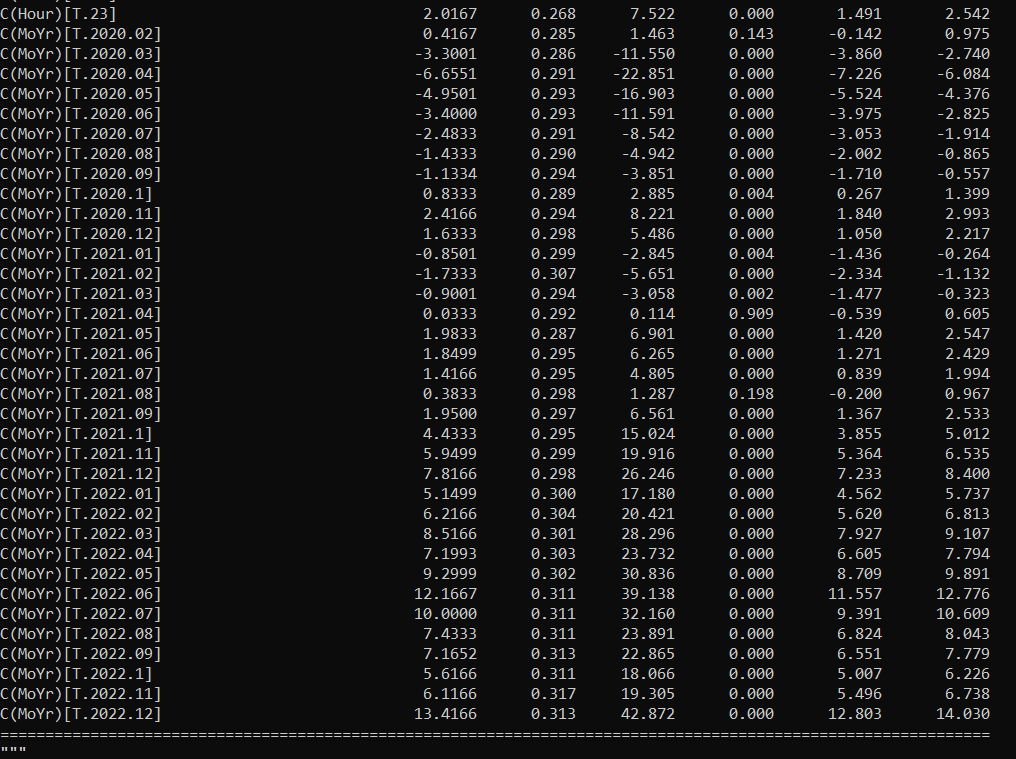You could of course make the model more complicated, e.g. look at spatial effects or incorporate other direct measures of capacity/people on duty. But here it is complicated enough for an illustrative blog post. January-2019 is the referent category month, and we can see some slight decreases in a few minutes around the start of the pandemic, but have been clearly been increasing at the median time fairly noticeably starting later in 2021.

As opposed to interpreting regression coefficients, I think it is easier to see model predictions. We can just make sample data points, here at noon over the different months, and do predictions over each different priority category:

``````# Predictions for different categories
hour = 12
prior_cat = [0,1,2]
oos = data.groupby(['PriorCat','MoYr'],as_index=False)['Hour'].size()
oos['Hour'] = 12
oos['Q50'] = res50.predict(oos)

print(oos[oos['PriorCat'] == '0'])
print(oos[oos['PriorCat'] == '1'])
print(oos[oos['PriorCat'] == '2'])``````

So here for priority 0, 130 has creeped up to 143.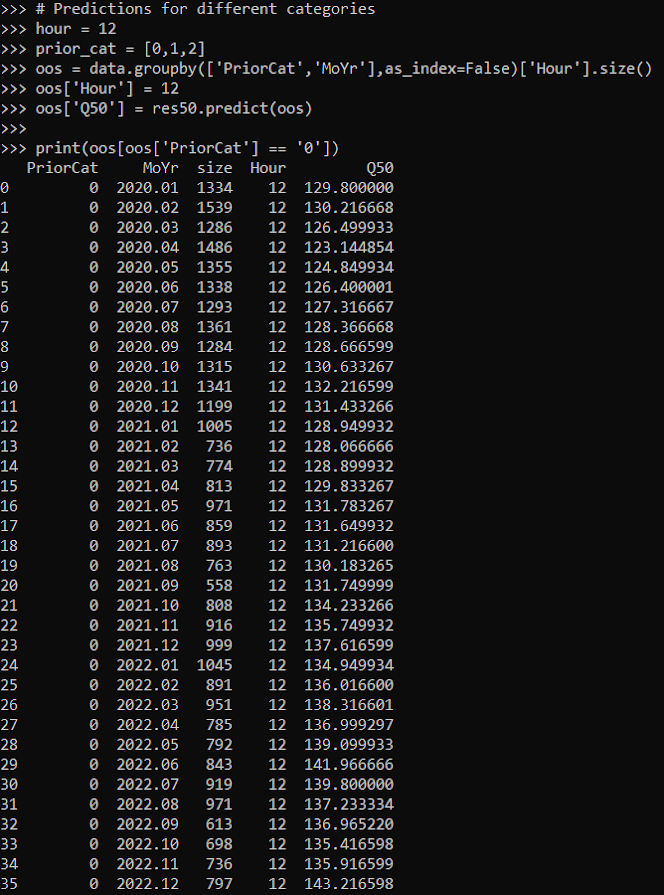And for priority 1, median times 35 to 49.Note that the way I estimated the regression equation, the increase/decrease per month is forced to be the same across the different priority calls. So, the increase among priority 2 calls is again around 13 minutes according to the model.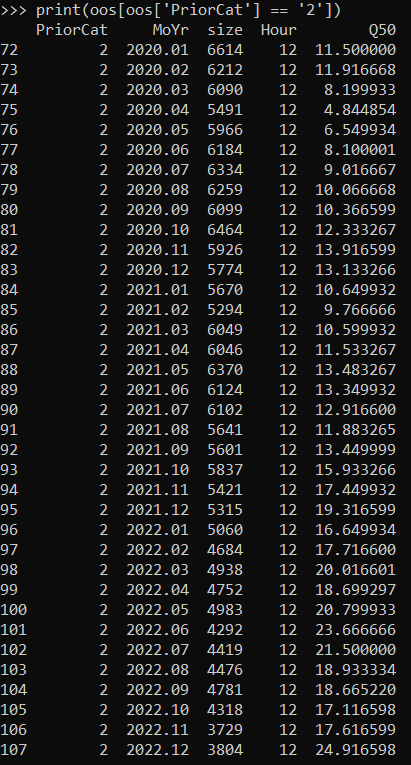But this assumption is clearly wrong. Remember my earlier “fast” and “slow” example, with only the slow calls increasing. That would suggest the distributions for the priority calls will likely have different changes over time. E.g. priority 0 may increase by alot, but priority 2 will be almost the same. You could model this in the formula via an interaction effect, e.g. something like `"minutes ~ C(PriorCat)*C(MoYr) + C(Hour)"`, but to make the computer spit out a solution a bit faster, I will subset the data to just priority 2 calls.

Here the power of quantile regression is we can look at different distributions. Estimating extreme quantiles is tough, but looking at the 10th/90th (as well as the median) is pretty typical. I do those three quantiles, and generate model predictions over the months (again assuming a call at 12).

``````# To save time, I am only going to analyze
# Priority 2 calls now
p2 = data[data['PriorCat'] == '2'].copy()
m2 = smf.quantreg("minutes ~ C(MoYr) + C(Hour)", p2)
oos2 = oos[oos['PriorCat'] == '2'].copy()

# loop over different quantiles
qlist = [0.1, 0.5, 0.9]
for q in qlist:
res = m2.fit(q=q)
oos2[f'Q_{q}'] = res.predict(oos2)

oos2``````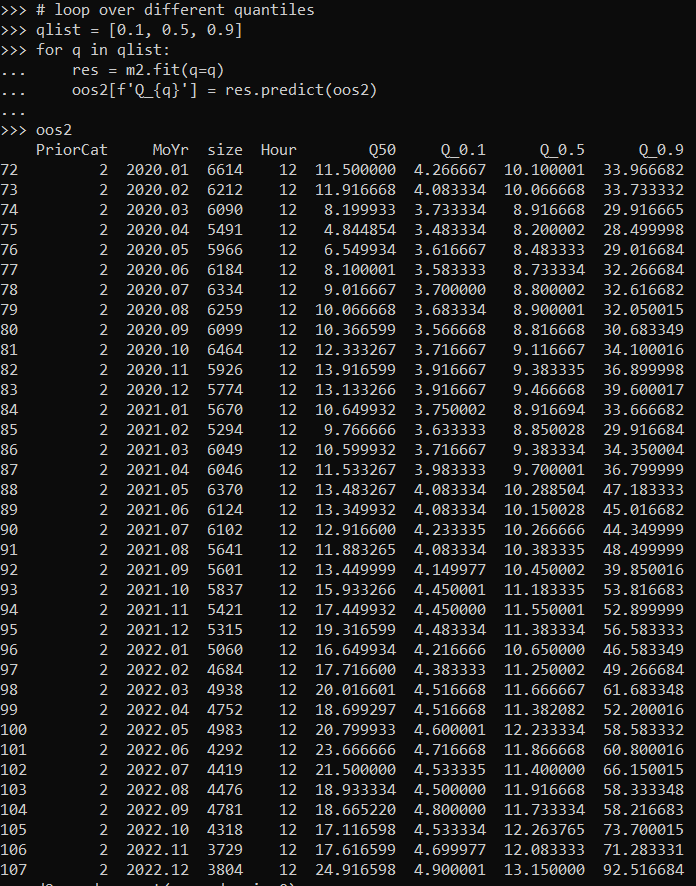So you can see my story about fast and slow calls plays out, although even when restricted to purportedly high risk calls. When looking at just priority 2 calls in New Orleans, the 10th percentile stays very similar over the period, although does have a slight increase from under 4 to almost 5 minutes. The 50th percentile has slightly more growth, but is from 10 minutes to 13 minutes. The 90th percentile though has more volatility – grew from 30 to 60 in small increases in 2022, and late 2022 has fairly dramatic further growth to 70/90 minutes. And you can see how the prior model that did not break out priority 0/1 calls changed this estimate for the left tail for the priority 2 left tail as well. (So those groups likely also had large shifts across the entire set.)

So my earlier scenario is overly simplistic, we can see some increase in the left tails of the distribution as well in this analysis. But, the majority of the increase is due to changes in the long right tail – calls that used to take less than 30 minutes are now taking 90 minutes to arrive. Which still likely has implications for satisfaction with police and reporting behavior, maybe not so much though with clearance or direct public safety.

No easy answers here in terms of giving internet advice to New Orleans. If working with NOLA, I would like to get estimates of officer capacity per shift, so I could incorporate into the quantile regression model that factor directly. That would allow you to precisely quantify how officer capacity impacts the distribution of response times. So not just “response times are going up” but “the decrease in capacity from A to B resulted in X increase in the 90th percentile of response times”. So if NOLA had goals set they could precisely state where officer capacity needed to be to have a shot of obtaining that goal.